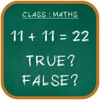UpdatedMathematics Fun
0 (0)
Pulled from iTunes
Wishlist
Mathematics Fun
Cody Keith
iPhone
Rating (Average):
0 (0)
Rating (Current):
0 (0)
Best free math practice game for everyone. Improve your mathematics with simple True False answer.
Solve maths equation with True False Puzzle Game.

Rules for the game as follow.
there are three Level Types are available:
1. Easy,
2. Medium,
3. Hard
Auto math equation or equations are generated like Addition, Subtraction, Multiplication.

User simple verified the answer is True or False and increase Score Card Board.

Simple Tap Tap App and improve your mathematics power using vedic maths and sharp your brain and become Maths genius.
What's new in v2.1